Signal ProcessingShowing results 51 to 60 of 69, on page 6 of 7 Fourier Transform Pairs Fourier Transform Properties Laplace Transform Pairs Laplace Transform Properties Z Transform Pairs Z Transform Properties
 DESCRIPTION EQUATION Z-transform involving the unit step function and an exponential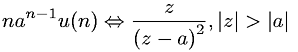Z-transform involving the unit step function and an exponential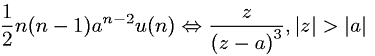Z-transform involving the unit step function and an exponential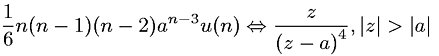Z-transform involving the unit step function and an exponential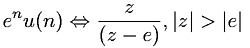Z-transform involving the unit step function and cosine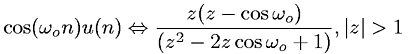Z-transform involving the unit step function and cosine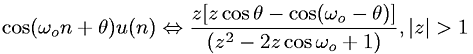Z-transform involving the unit step function and hyperbolic cosine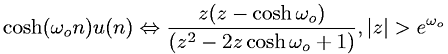Z-transform involving the unit step function and hyperbolic sine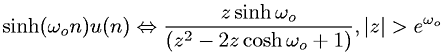Z-transform involving the unit step function and sine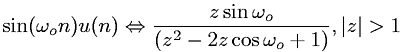Z-transform involving the unit step function and sine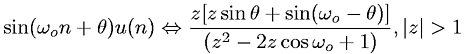Go to page: 1  2  3  4  5  6  7